# 4. Coloring and Mapping¶

In these notes you will learn:

• How to specify RGB colors.
• How to use grayscale.
• How to set the background, fill, and stroke colors in Processing.
• How to use variables to store frequently-used colors.
• How to use the map function.

## 4.1. Introduction¶

The art and science of color is a huge topic, and this note is a brief and practical introduction to RGB color as used in Processing.

## 4.2. RGB Color¶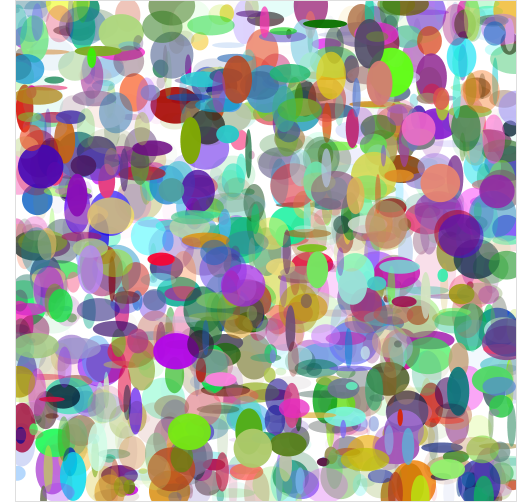RGB is short for “red, green, blue”: all RGB colors are combinations of different amounts of red, green, and blue.

For example, the color orange is written as (255, 165, 0) in RGB. We call (255, 165, 0) an RGB triplet because it consists of three numbers. RGB triplets always have the form (red, green, blue), where the numbers red, green, and blue represent the intensity of that color. So orange, which is (255, 165, 0), is a combination of red at intensity 255, green at intensity 165, and blue at intensity 0 (i.e. there is no blue in orange).

For each red/green/blue value, the minimum intensity is 0, and the maximum intensity is 255. Thus each of red, green, and blue have 256 intensity levels, which together can name exactly 16,777,216 different colors, i.e. over 16 million unique colors. On a good color monitor this is enough to display photo-realistic images.

Why 16,777,216 different colors? In the RGB triple (r, g, b), each of r, g, and b can take on one 256 different values. Thus, there are 256 * 256 * 256 = 256^3 = 16,777,216 different RGB triples.

Note

If you are curious, here is an image that shows all 16,777,216 RGB colors, one color per pixel. It’s a 4096 x 4096 image and is over 34 megabytes in size.

It’s useful to know a few basic RGB colors. Black is (0, 0, 0) and can be thought of as the absence of any color. In contrast, white is (255, 255, 255).

Pure red is (255, 0, 0), and (128, 0, 0) is a darker shade of red; it’s darker because (128, 0, 0) is closer to black. Similarly, pure green is (0, 255, 0) and pure blue is (0, 0, 255).

Note

One way to get more RGB colors is to look at one of the many RGB color charts on the web. For example, this page shows you numerous colors and give their names and RGB triplets.

## 4.3. Grayscale¶

A number of Processing functions support grayscale color. Grayscale is important when you need to display an image somewhere that doesn’t support full RGB. For instance, many newspapers are grayscale, and so must convert color photographs to grayscale. In RGB, any triplet of the form (c, c, c) specifies a shade of gray, and is considered to be a grayscale color. Since there are 256 different possible values for c, these grayscale colors have 256 different shades: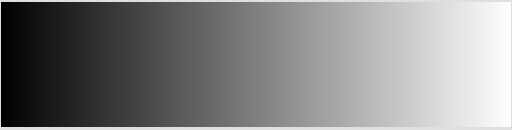Grayscale need not use the color gray. Sometimes different shades of a color can be used in grayscale. For instance, here are three different grayscales, using 256 shades of red, green, and blue respectively: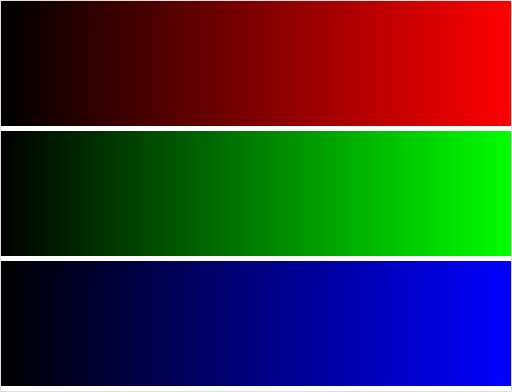## 4.4. Setting the Background Color¶

To set the background color of the drawing window, uses the background function, e.g.:

```background(255, 165, 0);  // orange
```

To set the background to be a shade of gray you could do this:

```background(128, 128, 128);
```

Or, equivalently, this:

```background(128);
```

## 4.5. Setting the Fill Color¶

Shapes, such as ellipses and rectangles, can have their interiors filled with any color using the fill function. For example:

```fill(255, 165, 0);  // orange fill
ellipse(100, 100, 60, 40);

fill(100, 100, 100); // gray fill
rect(100, 100, 60, 40);
```

The fill color is always the same as the last call to fill.

For grayscale colors you can write fill(c), or fill(c, c, c) if you like typing.

You can also specify that a shape have no fill color at all, i.e. that it have a completely transparent interior that will let whatever is underneath show through. To do this, use the noFill() function, e.g.:

```noFill();
ellipse(100, 100, 60, 40);
```

## 4.6. Setting the Stroke Color (and size)¶

Shapes also have an edge with a color that can be different from its fill color. The stroke function set the color of the edge, e.g.:

```fill(255, 165, 0);  // orange fill
stroke(0, 50, 0);   // dark green stroke
ellipse(100, 100, 60, 40);
```

If you don’t want an edge on a shape, then use noStroke(). As with the other color-setting functions, calling stroke(c) will set the stroke to be the grayscale color (c, c, c).

You can set the thickness of the stroke using the strokeWeight function, e.g.:

```strokeWeight(10);
```

## 4.7. Color Variables¶

Remembering and writing RGB triplets is rather tedious, and so it is often convenient to store colors in variables. For example:

```color orange = color(255, 165, 0);
```

This statement creates a new variable named orange that labels a color object. In a Processing program we would use it like this:

```color orange = color(255, 165, 0);
color dark_green = color(0, 50, 0);
color white = color(255, 255, 255);

void setup() {
size(500, 500);
}

void draw() {
background(white);
fill(orange);
stroke(dark_green);
ellipse(100, 100, 60, 40);
}
```

Notice that we define the three color variables outside of any function. That way they can be used by any function that needs them: they are global variables.

Using color variables has a couple of advantages. First, they make the program more readable. When you see the statement fill(orange) you have a pretty good idea about what it does. In contrast, the statement fill(255, 165, 0) is not as readable. Second, it easier to change the colors later. For instance, suppose you decide that you want your green to be darker, and so all you need to do is change its definition in one place:

```color dark_green = color(0, 30, 0);   // 30 used to be 50
```

Everywhere in your program where dark_green is used will now have this new color.

## 4.8. Transparency¶

Processing also lets you specify how transparent a color is. For example, in this program the moving rectangle has a transparency value of 100:

```color orange = color(255, 165, 0);
color dark_green = color(0, 50, 0);
color white = color(255, 255, 255);

void setup() {
size(255, 255);
}

void draw() {
background(white);
fill(orange);
ellipse(255/2, 255/2, 255, 255);
fill(255, 0, 0, 100);
rect(mouseX, mouseY, 128, 128);
}
```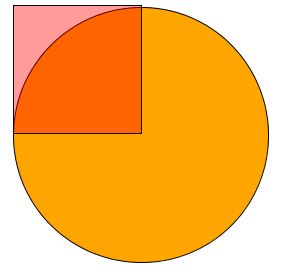The rectangle acts a bit like red cellophane, i.e. you can see through to whatever is underneath. By changing 100 to different values you can get different levels of transparency.

Transparent colors have the format (r, g, b, alpha), where the so-called alpha-value is, like the others, a number from 0 (totally transparent) to 255 (totally opaque).

## 4.9. The Processing IDE Color Selector¶

If you are using the programming editor that comes with Processing, then you may want to use the color selector tool that comes with it. To use it, open the Tools menu and choose “Color Selector”. A window with a color wheel and various boxes should appear. Click on any color in the wheel and the RGB values for that color will be instantly displayed.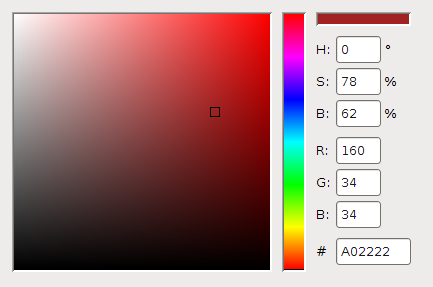Notice that in addition to RGB color, the selector also supports HSB color codes, which can sometimes be more convenient than RGB.

## 4.10. More Color¶

We’ve just scratched the surface of color in Processing, but it will suffice for most of the programs we’ll write in this course. The Processing documentation lists many other color-related functions, and they are a good start for learning more about colors.

## 4.11. Example: Colors and Arithmetic¶

It’s often interesting to set colors automatically. For instance, lets modify the program from the previous section to draw circles whose fill color is a shade of gray that depends upon mouseY:

```void setup() {
size(500, 500);
noStroke();
}

void draw() {
fill(mouseY);   // not so good!
ellipse(mouseX, mouseY, 50, 50);
}
```

This program simply sets the fill color of the ellipse to be mouseY. The results are not quite what we want: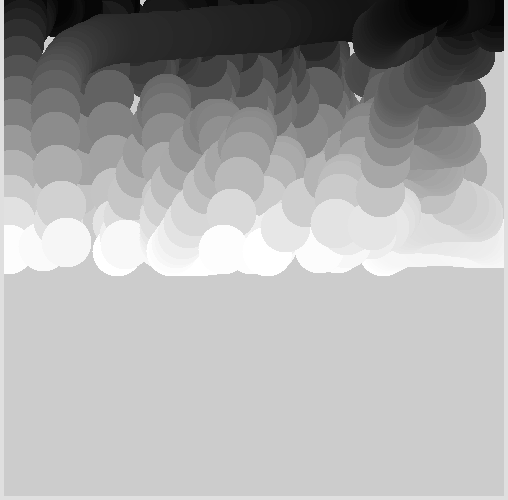As you can see, circles are drawn only on the top half the screen. That’s not what we want: we want circles to be drawn anywhere on the screen.

Why is this happening? The problem is that the range of legal values for mouseY is bigger than the range of legal values for fill. Specifically, mouseY ranges from 0 to 500 (the width of the screen), but fill values only range from 0 to 255.

So we can’t just use mouseY as the fill value. Instead, we need to create a new expression, based on mouseY, that returns values in the range 0 to 255.

The trick is to use this expression: 255 * (mouseY / 500). The sub-expression mouseY / 500 is guaranteed to be in the range 0 to 1 because the maximum possible value of mouseY is 500 (and the smallest possible value is 0). Thus, multiplying mouseY / 500 by 255 gives a value that is in the range 0 to 255, which is what fill needs:

```void draw() {
fill(256 * mouseY / 500);   // good!
ellipse(mouseX, mouseY, 50, 50);
}
```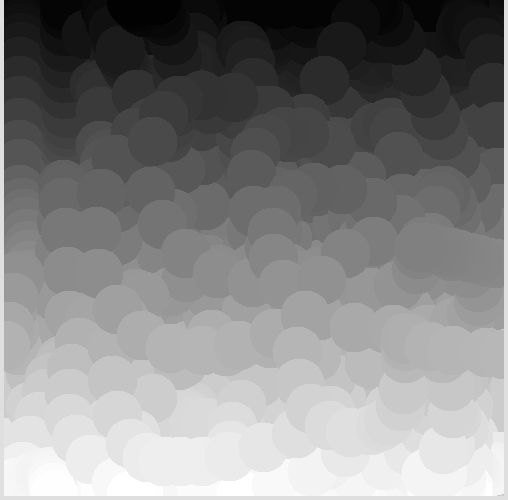## 4.12. Using the map Function¶

Shrinking and stretching numbers like this is so common in graphics programming that Processing provides a helper function to do it for you. The map(x, low1, high1, low2, high2) function converts x from the source range [low1, high1] to a new value in the target range [low2, high2]. The key fact is that the new value is at proportionally the same position in the target range as x is in the source range.

For example, suppose you have a point 25% of the way between 10 and 20 (i.e. the point 12.5):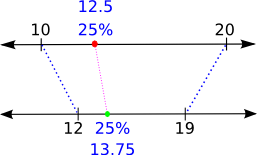Then map(12.5, 10, 20, 12, 19) returns the point 13.75, which is exactly 25% of the way between 12 and 19.

As another example, in the program from the previous section the first range is [0, 500 * 500], i.e. the range of possible values for mouseX * mouseY. The second range is [0, 255], the range of all legal grayscale colors. We can associate each number in the range [0, 500 * 500] with a number in [0, 255] using this function call:

```map(mouseX * mouseY, 0, 500 * 500, 0, 255)
```

This always returns a number in the target range, i.e. a number from 0 to 255. And using this function we can choose colors based on the mouse position like this:

```void setup() {
size(500, 500);
}

void draw() {
noStroke();
fill(map(mouseX * mouseY, 0, 500 * 500, 0, 255));
ellipse(mouseX, mouseY, 50, 50);
}
```

Since map is so useful, lets look at one final example. This time, lets draw an ellipse whose diameters are always between 25 and 100, and change in proportion to the mouse location:

```color orange = color(255, 165, 0);
color white = color(255, 255, 255);

void setup() {
size(500, 500);
noStroke();
smooth();
}

void draw() {
background(white);
fill(orange);
ellipse(mouseX, mouseY, map(mouseX, 0, 500, 25, 100),
map(mouseY, 0, 500, 25, 100));
}
```

Since the screen is 500 by 500, we know that mouseX (and mouseY) must be in the range [0, 500]. The legal diameter values are in the range [25, 100], and so map(mouseX, 0, 500, 25, 100) returns a value in [25, 100] that corresponds to the same location as mouseX in the range [0, 500].

## 4.13. Questions¶

1. What does RGB stand for?

2. In a Processing RGB triple (r, g, b), what are the smallest and largest possible values for r, g, and b?

3. About how many different colors can RGB specify?

4. Explain the difference between fill color and stroke color for a shape such as an ellipse or rectangle.

5. Explain the difference between noFill() and fill(0, 0, 0).

6. In the following program, the color of a circle and the background change according to the position of the mouse pointer:

```void setup() {
size(255, 255);
}

void draw() {
background(mouseX, mouseY, 0);
fill(255 - mouseX, 255 - mouseY, 0);
noStroke();
ellipse(255/2, 255/2, 255/2, 255/2);
}
```

At what point must the mouse pointer be for the circle to be invisible, i.e. the same color as the background?

7. Give two different reasons why color variables are useful in Processing.

8. Why are color variables typically defined outside of any function?

9. In the Processing color (r, g, b, alpha), what does alpha represent?

10. Explain how the map function works. Use an example with specific numbers to make your explanation concrete.

## 4.14. Programming Questions¶

1. Write a program that draws a purple square with a yellow edge at the mouse pointer. Make the width and height of the square equal to (mouseX + mouseY) / 5, and use strokeWeight to give the edge a thickness of at least 10.

2. Modify the previous program so that, in addition to what it already does, the color of the square’s interior is based on mouseX + mouseY. Use the map function to convert mouseX + mouseY into a legal RGB color.

3. Write a program that draws an ellipse centered at the mouse pointer and its fill color set to be proportional to mouseX * mouseY.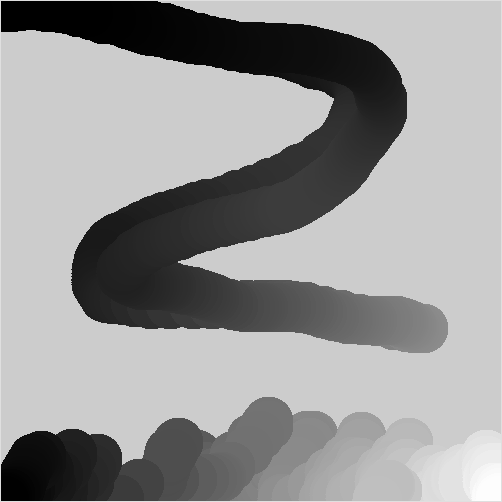4. Write a program that draws four differently colored triangles on the screen. The base of each triangle should be one of the four edges of the screen, and one point of each triangle should follow the mouse pointer.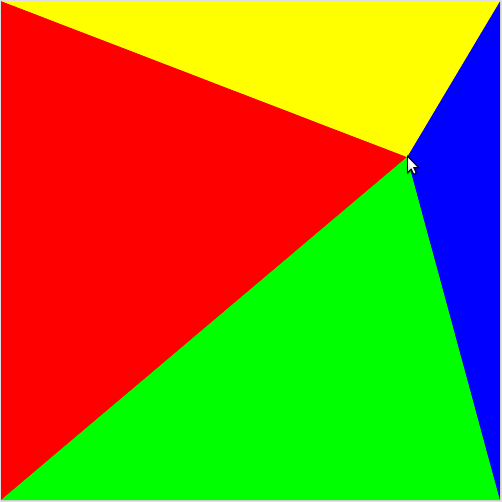As the pointer moves, the four triangle change shape. There bases always stay attached to the screen edges.